# KR101318519B1 - Ofdm demodulation device and method - Google Patents

## Info

Publication number
KR101318519B1
KR101318519B1 KR1020087019480A KR20087019480A KR101318519B1 KR 101318519 B1 KR101318519 B1 KR 101318519B1 KR 1020087019480 A KR1020087019480 A KR 1020087019480A KR 20087019480 A KR20087019480 A KR 20087019480A KR 101318519 B1 KR101318519 B1 KR 101318519B1
Authority
KR
South Korea
Prior art keywords
signal
ofdm
carrier frequency
offset amount
frequency offset
Prior art date
Application number
KR1020087019480A
Other languages
Korean (ko)
Other versions
KR20080107368A (en
Inventor
다꾸야 오까모또
다꾸 야마가따
다까히로 오까다
Original Assignee
소니 주식회사
Priority date (The priority date is an assumption and is not a legal conclusion. Google has not performed a legal analysis and makes no representation as to the accuracy of the date listed.)
Filing date
Publication date
Priority to JP2006032831A priority Critical patent/JP4419969B2/en
Priority to JPJP-P-2006-00032831 priority
Application filed by 소니 주식회사 filed Critical 소니 주식회사
Priority to PCT/JP2007/052034 priority patent/WO2007091563A1/en
Publication of KR20080107368A publication Critical patent/KR20080107368A/en
Application granted granted Critical
Publication of KR101318519B1 publication Critical patent/KR101318519B1/en

• 239000000969 carriers Substances 0.000 claims abstract description 142
• 230000005540 biological transmission Effects 0.000 claims abstract description 42
• 230000001186 cumulative Effects 0.000 claims abstract description 22
• 230000000051 modifying Effects 0.000 claims abstract description 20
• 238000000034 methods Methods 0.000 claims description 14
• 230000001131 transforming Effects 0.000 claims 3
• 230000018109 developmental process Effects 0.000 description 1

## Images

••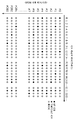•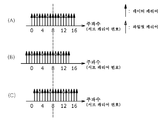•••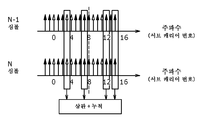•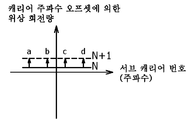••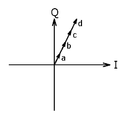•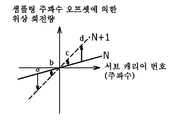•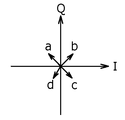••••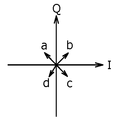•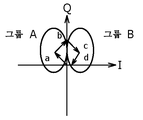••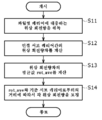•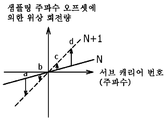•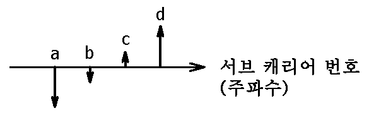•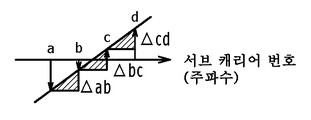•## Classifications

• HELECTRICITY
• H04ELECTRIC COMMUNICATION TECHNIQUE
• H04LTRANSMISSION OF DIGITAL INFORMATION, e.g. TELEGRAPHIC COMMUNICATION
• H04L27/00Modulated-carrier systems
• H04L27/26Systems using multi-frequency codes
• H04L27/2601Multicarrier modulation systems
• H04L27/2647Arrangements specific to the receiver
• H04L27/2655Synchronisation arrangements
• H04L27/2657Carrier synchronisation
• HELECTRICITY
• H04ELECTRIC COMMUNICATION TECHNIQUE
• H04LTRANSMISSION OF DIGITAL INFORMATION, e.g. TELEGRAPHIC COMMUNICATION
• H04L27/00Modulated-carrier systems
• H04L27/26Systems using multi-frequency codes
• H04L27/2601Multicarrier modulation systems
• H04L27/2647Arrangements specific to the receiver
• H04L27/2655Synchronisation arrangements
• H04L27/2668Details of algorithms
• H04L27/2673Details of algorithms characterised by synchronisation parameters
• H04L27/2675Pilot or known symbols
• HELECTRICITY
• H04ELECTRIC COMMUNICATION TECHNIQUE
• H04LTRANSMISSION OF DIGITAL INFORMATION, e.g. TELEGRAPHIC COMMUNICATION
• H04L27/00Modulated-carrier systems
• H04L27/26Systems using multi-frequency codes
• H04L27/2601Multicarrier modulation systems
• H04L27/2602Signal structure
• H04L27/261Details of reference signals
• HELECTRICITY
• H04ELECTRIC COMMUNICATION TECHNIQUE
• H04LTRANSMISSION OF DIGITAL INFORMATION, e.g. TELEGRAPHIC COMMUNICATION
• H04L5/00Arrangements affording multiple use of the transmission path
• H04L5/0001Arrangements for dividing the transmission path
• H04L5/0003Two-dimensional division
• H04L5/0005Time-frequency
• H04L5/0007Time-frequency the frequencies being orthogonal, e.g. OFDM(A), DMT
• HELECTRICITY
• H04ELECTRIC COMMUNICATION TECHNIQUE
• H04LTRANSMISSION OF DIGITAL INFORMATION, e.g. TELEGRAPHIC COMMUNICATION
• H04L5/00Arrangements affording multiple use of the transmission path
• H04L5/003Arrangements for allocating sub-channels of the transmission path
• H04L5/0048Allocation of pilot signals, i.e. of signals known to the receiver

## Abstract

The present invention provides an OFDM demodulation device for demodulating an orthogonal frequency division multiplex (OFDM) signal whose transmission symbol is generated by orthogonal modulation by dividing information for a plurality of subcarriers within a predetermined band, wherein the carrier frequency offset is detected. The circuit 20 is provided. The carrier frequency offset detection circuit divides a subcarrier at a position where a TMCC signal, an AC signal, or a CP signal, which is determined according to a standard, into a plurality of groups, assuming a specific offset amount, and phases from the immediately preceding OFDM symbol. Map the square of the rotation amount to the complex plane and convert it into a rotation vector, accumulate and add the rotation vector for each group to calculate the absolute value of the cumulative addition value, and calculate the absolute value of the cumulative addition value calculated for each group for all groups. The addition absolute value is calculated by adding, and the offset amount assumed when the maximum addition absolute value is obtained is taken as the carrier frequency offset amount.
Carrier frequency, transmission symbol, subcarrier, carrier frequency offset amount, pilot carrier, OFDM symbol, phase rotation amount

## Description

OPDM demodulation device and method {OFDM DEMODULATION DEVICE AND METHOD}

The present invention relates to an OFDM demodulation device and method for demodulating an orthogonal frequency division multiplex (OFDM) signal.

This application claims priority based on Japanese Patent Application No. 2006-32831 for which it applied on February 9, 2006 in Japan, This application is integrated in this application by reference.

As a method of transmitting a digital signal, a modulation method called an Orthogonal Frequency Division Multiplexing (OFDM) method (hereinafter, referred to as an OFDM method) is used. In the OFDM method, a plurality of orthogonal subcarriers (subcarriers) are set in a transmission band, and data is assigned to the amplitude and phase of each subcarrier by means of phase shift keying (PSK) or quadrature amplitude modulation (QAM) to perform digital modulation. That's the way it is.

Since the OFDM system divides the transmission band into a plurality of subcarriers, the band per subcarrier becomes narrower and the modulation rate is slowed, but the total transmission rate is not changed from the conventional modulation method. . In addition, the OFDM scheme is characterized in that the symbol rate is slowed down because a large number of subcarriers are transmitted in parallel, so that the time length of the multipath relative to the time length of the symbol can be shortened, thereby making it difficult to be subjected to multipath interference. Have

In addition, in the OFDM method, since data is allocated to a plurality of subcarriers, an Inverse Fast Fourier Transform (IFFT) calculation circuit that performs inverse Fourier transform at the time of transmission, and a FFT (Fast Fourier Transform) that performs Fourier transform at the time of reception. By using an arithmetic circuit, a transmission and reception circuit can be configured.

From the above characteristics, the OFDM method is often applied to terrestrial digital broadcasting which is strongly affected by multipath interference. Examples of terrestrial digital broadcasting employing such an OFDM system include DVB-T (Digital Video Broadcasting-Terrestrial), ISDB-T (Integrated Services Digital Broadcasting-Terrestrial), and ISDB-T SB (ISDB-T Sound Broadcasting). And the like.

As shown in Fig. 1, an OFDM transmission symbol (hereinafter, referred to as an OFDM symbol) is a valid symbol that is a signal period during which an IFFT operation is performed at the time of transmission, and a waveform of a part of the latter half of the valid symbol is copied as it is. Consists of protected guard intervals. The guard period is set in the first half of the OFDM symbol. In the OFDM scheme, such guard intervals are set, thereby allowing intersymbol interference by multipath, thereby improving multipath immunity.

In the OFDM system, it is prescribed that a plurality of OFDM symbols as described above are collected to form a transmission unit called one OFDM frame. For example, in the ISDB-T standard, one OFDM frame is formed of 204 OFDM symbols. In the OFDM method, for example, a scattered pilot (SP) signal (hereinafter, referred to as an SP signal) used for estimating transmission line characteristics, a transmission parameter, or the like is included on the basis of this OFDM frame unit. The insertion position of the TMCC (Transmission and Multiplexing Configuration Control) / AC (Auxiliary Channel) signal is determined.

2 shows an arrangement pattern of an SP signal and an TMCC / AC signal in an OFDM frame employed in the ISDB-T standard. The SP signal is subjected to BPSK (Binary Phase Shift Keying) modulation and inserted in one ratio in 12 subcarriers in the subcarrier direction (frequency direction). In addition, the SP signal is inserted in four OFDM symbols in one ratio with respect to the same subcarrier in the OFDM symbol direction (time direction). On the other hand, the TMCC / AC signal is differentially BPSK modulated and inserted into a plurality of predetermined subcarriers. The TMCC / AC signal is inserted in all OFDM symbols of the same subcarrier in the OFDM symbol direction (time direction).

In the following description, a subcarrier into which a TMCC / AC signal is inserted is referred to as a pilot carrier, and a subcarrier into which a normal data signal is inserted is referred to as a data carrier.

By the way, in the OFDM receiver which receives the OFDM signal which uses the above-mentioned OFDM symbol as a transmission unit, the original carrier arrangement as shown in FIG. 3A is based on the carrier frequency offset, and FIG. As shown in FIG. 3, there may be a shift in the low frequency direction or a shift in the high frequency direction as shown in FIG. 3C. In addition, in FIG.3 (A)-FIG.3 (C), only 17 subcarriers are shown for simplicity. Therefore, in the OFDM receiver, it is necessary to detect the carrier frequency offset amount and remove the influence of the carrier frequency offset.

Conventionally, as a method of detecting the carrier frequency offset amount with subcarrier precision, there is a use of correlation between adjacent OFDM symbols of the above-described pilot carriers. For details of this method, see Kenichiro Hayashi, "Development of OFDM Element Technology," Technical Information Report, ITE Technical Report, Vol.23, No.28, pp.25-30, Mar, 1999. The procedure of this carrier frequency offset amount detection is demonstrated with reference to the flowchart shown in FIG.

First, in step S101, the amount of phase rotation from 1 OFDM symbol before each subcarrier of the orthogonal demodulation is calculated. In the ideal reception state, the phase rotation amount is 0 degrees or 180 degrees because of the differential BPSK modulation in the pilot carrier, and the phase rotation amount becomes random because, for example, 64 QAM modulation is performed in the data carrier. In addition, in order to avoid the phase rotation amount in the pilot carrier becoming two types of 0 degree and 180 degree, square processing is performed before calculating the phase rotation amount so that all are brought close to the 0 degree side.

Next, in step S102, the hypothesis offset amount is defined as k, and k is set to the minimum value of the search range. The hypothetical offset amount k means that the k subcarriers are offset from the position centered on the circuit. Subsequently, at step S103, it is determined whether or not the hypothesis offset amount k is the maximum value of the search range, and if it is not the maximum value, the process proceeds to step S104 and, if the maximum value is the maximum value, the process proceeds to step S107.

In step S104, the phase rotation amount of the pilot carrier position determined by specification is assumed when k subcarriers are offset, and in step S105, each phase rotation amount is mapped to a circumference of a fixed radius on the complex plane and rotated. Convert them to vectors and add them all cumulatively. In step S106, k is incremented by one, and the flow returns to step S103.

In step S107, the absolute value of the cumulative addition value is determined, and the absolute value is found to be the maximum. In step S108, the assumed offset amount k when the maximum absolute value is obtained is output as the original carrier frequency offset amount. do. When the hypothesis offset amount k coincides with the original carrier frequency offset amount, the absolute value increases because only the rotation vector of the pilot carrier is accumulated, but when the assumption offset amount k does not coincide with the original carrier frequency offset amount, Since the rotation vector of the data carrier is added cumulatively, it is based on the cancellation and the absolute value of the data carrier.

For example, when the carrier frequency offset amount is -2 and k = -2, since the correlation between the OFDM symbols of the pilot carriers is obtained as shown in Fig. 5A, the absolute value of the cumulative addition value is obtained. Becomes large, but when the carrier frequency offset amount is -2 and k = +1, since the correlation between OFDM symbols of the data carrier is obtained as shown in Fig. 5B, the absolute value of the cumulative addition value is obtained. Becomes smaller.

<Start of invention>

[PROBLEMS TO BE SOLVED BY THE INVENTION]

Although the ideal reception state was assumed in the above-described detection method of the carrier frequency offset amount, various offsets such as the sampling frequency offset as well as the carrier frequency offset overlap in the actual reception environment.

Here, when there is a carrier frequency offset, as shown in Fig. 6A, the same amount of phase rotation as the entire subcarriers is applied between symbol N and symbol N + 1. Therefore, when the phase rotation amounts of the pilot carriers represented by a, b, c, and d in the figure are mapped to a complex plane and converted into rotation vectors as shown in Fig. 6B, all of them are cumulatively added. As shown in Fig. 6C, since the absolute value of the cumulative addition value increases, the magnitude and difference of the absolute value of the cumulative addition value when cumulatively adding the rotation vector of the data carrier is generated. As described above, the carrier frequency The offset can be detected.

However, when the sampling frequency offset exists, as shown in Fig. 7A, the amount of phase rotation in proportion to the subcarrier number is applied between the symbol N and the symbol N + 1. Therefore, when the phase rotation amounts of the pilot carriers represented by a, b, c, and d in the figure are converted into rotation vectors by mapping them to the complex plane as shown in Fig. 7B, all of them are cumulatively added. As shown in Fig. 7C, the cumulative addition value is canceled. When the distribution of the phase rotation amount is small, there are few canceling components. Therefore, the magnitude and difference of the absolute value of the cumulative addition value when the rotation vector of the data carrier is cumulatively added causes a carrier frequency offset to be detected. When the subcarriers are distributed from -π to + π, the cumulative addition value is completely canceled, and as a result, the carrier frequency offset cannot be detected.

Therefore, conventionally, in order to avoid such a problem, since the amount of sampling frequency offset must be detected beforehand and the influence of a sampling frequency offset must be removed, there existed a problem that an additional circuit and processing time are needed.

SUMMARY OF THE INVENTION The present invention has been proposed in view of the above-described conventional situation, and provides an OFDM demodulation device and method capable of detecting a carrier frequency offset amount without detecting a sampling frequency offset amount in advance. Is in.

One embodiment of an OFDM demodulation device according to the present invention is an OFDM demodulation device that demodulates an orthogonal frequency division multiplex (OFDM) signal, and orthogonally demodulates the OFDM signal using a carrier signal of a specific frequency, thereby performing baseband OFDM. A carrier frequency that is a deviation amount of the center frequency of the OFDM signal of the baseband based on the correlation between the orthogonal demodulation circuit for generating the signal and the transmission symbol of the subcarrier into which the predetermined signal is inserted among the OFDM signals in the frequency domain. A carrier frequency offset detection circuit for detecting the offset amount with subcarrier precision, and a frequency control unit for controlling the frequency of the carrier signal in accordance with the carrier frequency offset amount, and the carrier frequency offset detection circuit assumes a specific offset amount. The subcarrier at the position where the predetermined signal is inserted into a plurality of groups And a correlation value with adjacent transmission symbols is calculated for each group, the correlation value is added to all groups, and the offset amount assumed when the maximum addition value is obtained is used as the carrier frequency offset amount. do.

In addition, an embodiment of the OFDM demodulation method includes an orthogonal frequency division multiplex (OFDM) signal in which transmission symbols generated by orthogonal modulation of information is divided and transmitted for a plurality of subcarriers within a predetermined band. An OFDM demodulation method comprising: an orthogonal demodulation step of performing orthogonal demodulation of the OFDM signal using a carrier signal of a specific frequency, and a Fourier transform of the baseband OFDM signal in units of transmission symbols Fourier transform process for generating an OFDM signal in the frequency domain, and a subcarrier into which a Transmission and Multiplex Configuration (Control) signal, an AC (Auxiliary Channel) signal, or a Continuous Pilot (CP) signal is inserted among OFDM signals in the frequency domain. Based on the correlation between the transmitted symbols of the A carrier frequency offset detection step of detecting the frequency offset amount with subcarrier precision, and a frequency control step of controlling the frequency of the carrier signal in accordance with the carrier frequency offset amount. Assuming that, the subcarrier at the position where the TMCC signal, the AC signal, or the CP signal is inserted is divided into a plurality of groups, a correlation value with adjacent transmission symbols is calculated for each group, and the correlation value is applied to all groups. And the offset amount assumed when the maximum addition value is obtained as the carrier frequency offset amount.

According to the OFDM demodulation device and method to which the present invention is applied, even if a sampling frequency offset exists, the carrier frequency offset amount can be detected without detecting the sampling frequency offset amount in advance.

Further objects of the present invention and specific advantages obtained by the present invention will become more apparent from the embodiments described below with reference to the drawings.

1 is a diagram for explaining an OFDM signal, an OFDM symbol, a valid symbol, and a guard interval.

2 is a diagram for explaining an insertion position of an SP signal and a TMCC / AC signal in an OFDM signal.

3A to 3C are diagrams for explaining a carrier frequency offset.

4 is a flowchart for explaining a procedure of conventional carrier frequency offset amount detection.

5A and 5B are diagrams for explaining the carrier frequency offset amount and the hypothesis offset amount.

6A to 6C are diagrams for explaining the cumulative addition value of the phase rotation amount for each subcarrier and the phase rotation amount in the complex plane when the carrier frequency offset exists.

7A to 7C are diagrams for explaining the cumulative addition value of the phase rotation amount for each subcarrier and the phase rotation amount in the complex plane when a sampling frequency offset exists.

Fig. 8 is a block diagram of an OFDM receiver in this embodiment.

9A to 9C illustrate the concept of carrier frequency offset detection in an OFDM receiver.

Fig. 10 is a flowchart for explaining a procedure of carrier frequency offset amount detection in an OFDM receiver.

Fig. 11 is a flowchart for explaining a procedure for removing the influence of the sampling frequency offset from the phase rotation amount of a pilot carrier.

12A to 12D are diagrams for explaining an example of removing the influence of the sampling frequency offset from the phase rotation amount of the pilot carrier.

BEST MODE FOR CARRYING OUT THE INVENTION [

EMBODIMENT OF THE INVENTION Hereinafter, embodiment to which this invention is applied is described in detail, referring drawings.

First, a block diagram of the OFDM receiver according to the present embodiment is shown in FIG. In addition, in the description of the present specification, when the signal transmitted between blocks is a complex signal, a signal component is represented by a solid line, and when the signal transmitted between blocks is a real signal, the signal component is represented by a thin line.

As shown in FIG. 8, the OFDM receiver 1 includes an antenna 11, a frequency conversion circuit 12, a local oscillator 13, an A / D conversion circuit 14, and an orthogonal demodulation circuit. (15), carrier synchronization circuit 16, local oscillator 17, FFT calculation circuit 18, window regeneration circuit 19, carrier frequency offset detection circuit 20, equalization circuit 21 ).

The transmission wave transmitted from the OFDM transmission device is received by the antenna 11 of the OFDM reception device 1 and is supplied to the frequency conversion circuit 12 as an RF signal of the carrier frequency fc.

The RF signal received by the antenna 11 is frequency-converted to the IF signal of the intermediate frequency f IF by multiplying the carrier signal of the carrier frequency fc + f IF oscillated by the local oscillator 13 with the frequency conversion circuit 12. , A / D conversion circuit 14 is supplied. The IF signal is digitized by the A / D conversion circuit 14 and supplied to the orthogonal demodulation circuit 15.

The orthogonal demodulation circuit 15 orthogonally demodulates the digitized IF signal by using a carrier signal of the intermediate frequency f IF oscillated by the local oscillator 17 frequency controlled by the carrier synchronization circuit 16 to perform orthogonal demodulation. Outputs an OFDM signal. The baseband OFDM signal output from the orthogonal demodulation circuit 15 is a so-called time domain signal before FFT calculation. For this reason, hereinafter, the baseband signal before the FFT operation after orthogonal demodulation is called an OFDM time domain signal. As a result of orthogonal demodulation, the OFDM time domain signal is actually a complex signal including an axial component (I channel signal) and a virtual component (Q channel signal). The OFDM time domain signal output by the orthogonal demodulation circuit 15 is supplied to the carrier synchronization circuit 16, the FFT calculation circuit 18, and the window regeneration circuit 19.

The FFT calculation circuit 18 performs an FFT operation on the OFDM time domain signal to extract and output data orthogonally modulated on each subcarrier. The signal output from this FFT calculation circuit 18 is a so-called frequency domain signal after the FFT calculation. For this reason, hereinafter, a signal after the FFT operation is called an OFDM frequency domain signal.

The FFT arithmetic circuit 18 extracts a signal of a range of effective symbol lengths, for example, 2048 samples, from one OFDM symbol, i.e., extracts a range of guard intervals from one OFDM symbol, excluding 2048 samples. An FFT operation is performed on a sample OFDM time domain signal. Specifically, the operation start position is any one of the positions from the boundary of the OFDM symbol to the end position of the guard interval. This calculation range is called the FFT window.

As described above, the OFDM frequency domain signal output from the FFT arithmetic circuit 18 is a complex signal composed of a real axis component (I channel signal) and a virtual axis component (Q channel signal) similarly to the OFDM time domain signal. This complex signal is a signal that is orthogonally amplitude modulated by, for example, a 16 QAM system, a 64 QAM system, or the like. The OFDM frequency domain signal is supplied to the carrier frequency offset detection circuit 20 and the equalization circuit 21.

The window regeneration circuit 19 delays an OFDM time domain signal for an effective symbol period, obtains a correlation between a guard interval portion and a signal to be a copy source of the guard interval, and based on the high correlation portion, The boundary position is calculated, and a window synchronization signal indicating the boundary position is generated. The window regeneration circuit 19 supplies the generated window synchronization signal to the FFT calculation circuit 18.

The carrier frequency offset detection circuit 20 detects the carrier frequency offset amount, which is the shift amount of the center frequency, with subcarrier accuracy based on the OFDM frequency domain signal, and transmits the detected carrier frequency offset amount to the carrier synchronization circuit 16. Feedback. The carrier synchronization circuit 16 controls the oscillation frequency of the local oscillator 17 based on the feedback amount of carrier frequency offset. After this, the carrier synchronization circuit 16 can detect the carrier frequency offset amount of subcarrier precision or more based on the OFDM time domain signal, and control the oscillation frequency of the local oscillator 17. The details of the carrier frequency offset detection circuit 20 will be described later.

The equalization circuit 21 removes the modulation component by removing the information component from the OFDM frequency domain signal, extracting only the SP signal, and obtaining the difference between the extracted SP signal and the reference SP signal. The SP signal from which the modulation component has been removed shows the transmission path characteristics of the subcarrier into which the SP signal is inserted. The equalization circuit 21 performs time direction interpolation processing and frequency direction interpolation processing on the SP signal from which the modulation component is removed, estimates transmission path characteristics of all subcarriers in the OFDM symbol, and uses the estimated transmission path characteristics. Phase equalization and amplitude equalization of the OFDM frequency domain signal are performed. The equalization circuit 21 outputs an OFDM frequency domain signal subjected to phase equalization and amplitude equalization to the outside.

Next, the carrier frequency offset detection circuit 20 described above will be described in detail.

As described above, when the sampling frequency offset exists, as shown in Fig. 9A, the amount of phase rotation proportional to the subcarrier number is applied between the symbol N and the symbol N + 1. Therefore, if the phase rotation amounts of the pilot carriers represented by a, b, c, and d in the figure are mapped to a complex plane as shown in Fig. 9B, converted into rotation vectors, and all of them are cumulatively added, The cumulative addition value is canceled so that the carrier frequency offset cannot be detected.

Therefore, the carrier frequency offset detection circuit 20 according to the present embodiment does not cumulatively add all of the rotation vectors based on the pilot carriers represented by a, b, c, and d in the figure, but FIG. As shown in the figure, for example, a and b are group A, and c and d are group B, and the rotation vectors are cumulatively added in the group. The absolute value of the cumulative addition value obtained for each group is added to all the groups. As a result, it is possible to prevent the cumulative addition value from canceling and the final absolute value to become small, thereby making it impossible to detect the carrier frequency offset.

Therefore, the procedure of carrier frequency offset amount detection in the carrier frequency offset detection circuit 20 is demonstrated with reference to the flowchart shown in FIG.

First, in step S1, the amount of phase rotation from 1 OFDM symbol before each subcarrier of the OFDM time domain signal is calculated. In the ideal reception state, the phase rotation amount is 0 degrees or 180 degrees because of the differential BPSK modulation in the pilot carrier, and the phase rotation amount becomes random because, for example, 64 QAM modulation is performed in the data carrier. In addition, in order to avoid the phase rotation amount in the pilot carrier becoming two types of 0 degree and 180 degree, square processing is performed before calculating the phase rotation amount so that all are brought close to the 0 degree side.

Next, in step S2, the hypothesis offset amount is defined as k, and k is set to the minimum value of the search range. The hypothetical offset amount k means that the k subcarriers are offset from the position centered on the circuit. Subsequently, at step S3, it is determined whether or not the hypothesis offset amount k is the maximum value of the search range. If not, the process proceeds to step S4, and when the maximum value is not the maximum value, the process proceeds to step S7.

In step S4, the phase rotation amount of the pilot carrier position determined by specification is assumed when k subcarriers are offset, and in step S5, each phase rotation amount is mapped to the circumference of a fixed radius on the complex plane and rotated. Convert to vectors and add them cumulatively per group. In step S6, k is incremented by one and the flow returns to step S3.

In step S7, the absolute value of the cumulative addition value is obtained for each group, and these are added to all the groups, and the maximum absolute value is found. In step S8, the assumption is made when the maximum addition absolute value is obtained. The offset amount k is output as the original carrier frequency offset amount. This means that when the hypothesis offset amount k coincides with the original carrier frequency offset amount, only the rotation vector of the pilot carrier is accumulated and added, so that the absolute value of the addition increases, but when it does not coincide with the original carrier frequency offset amount, Since the rotation vector of the data carrier is cumulatively added, it is based on the offset and the absolute value of the addition being small.

In this way, the carrier frequency offset detection circuit 20 divides the pilot carriers into a plurality of groups, obtains the absolute value of the cumulative addition value for each group, and adds them to all the groups. Thereby, even if a sampling frequency offset exists, it is possible to prevent the cumulative addition value from canceling and the final absolute value to become small, thereby making it impossible to detect the carrier frequency offset.

The number of groups when dividing the pilot carriers into a plurality of groups can be arbitrarily set. However, as the number of groups increases, the number of offset components decreases and the memory size increases, so it is desirable to optimize the number of groups for each system.

In addition, each group which divided pilot carriers does not necessarily need to be comprised by the adjacent subcarriers. However, the farther the subcarrier number is, the more the offset components when the sampling frequency offset exists, so it is preferable to integrate the adjacent subcarriers into the same group.

By the way, according to the above-described detection method of the carrier frequency offset, the carrier frequency offset amount can be detected without detecting the sampling frequency offset amount in advance, but if additional circuitry or processing time is allowed, The carrier frequency offset amount may be detected after the influence is removed.

The procedure for removing the influence of this sampling frequency offset will be described with reference to the flowchart shown in FIG.

First, in step S11, assuming that the k subcarriers are offset as described above, the phase rotation amount of the pilot carrier position determined in the standard is obtained, and in step S12, the phase rotation amount difference between adjacent pilot carriers is calculated. .

Subsequently, in step S13, the average value rot_ave of the phase rotation amount difference is calculated, and in step S14, the phase rotation amount of each pilot carrier is corrected based on the average value rot_ave of the phase rotation amount difference and the reference distance from the pilot carrier. do.

For example, consider a case where a sampling frequency offset exists and a phase rotation amount proportional to the subcarrier number is applied between the symbol N and the symbol N + 1 as shown in Fig. 12A. Although the phase rotation amount of the pilot carrier shown by a, b, c, and d in the figure is as shown in FIG. 12B, in steps S12 and S13, as shown in FIG. The phase rotation amount differences Δab, Δbc, and Δcd between adjacent pilot carriers are calculated, and the average value rot_ave = (Δab + Δbc + Δcd) / 3 is calculated. And in step S14, the phase rotation amount of each pilot carrier is correct | amended based on this average value rot_ave and the distance from the reference | standard pilot carrier. Based on the pilot carrier represented by a in the figure and the phase rotation amounts of the pilot carriers represented by a, b, c, and d in the figures are represented by a, b, c and d, respectively, the phase rotation amount a 'after correction ， B '， c' ， d 'is

a '= a

b '= b + rot_ave × 1

c '= c + rot_ave × 2

d '= d + rot_ave × 3

It is expressed as

After that, similarly to the above, the amount of phase rotation after correction is mapped to a circumference of a fixed radius on the complex plane, and converted into a rotation vector, and all of them are cumulatively added. In addition, in this method, since the influence of the sampling frequency offset is eliminated, it is not necessary to divide the pilot carriers into a plurality of groups as described above.

As mentioned above, although some embodiment of this invention was described, this invention is not limited only to embodiment mentioned above, Of course, a various change is possible in the range which does not deviate from the summary of this invention.

For example, in the above-described embodiment, the phase rotation amount of the subcarrier in which the TMCC signal or the AC signal is inserted is used when detecting the carrier frequency offset, but the present invention is not limited thereto, but a CP (Continual Pilot) signal is inserted. The amount of phase rotation of the subcarrier may be used.

## Claims (7)

1. An OFDM demodulation device for demodulating an orthogonal frequency division multiplex (OFDM) signal,
Orthogonal demodulation means for orthogonal demodulating the OFDM signal using a carrier signal of a specific frequency to generate an OFDM signal of baseband;
Based on the correlation between the transmission symbols of the subcarrier into which the predetermined signal is inserted among the OFDM signals in the frequency domain, the carrier frequency offset amount which is the shift amount of the center frequency of the OFDM signal of the baseband is detected with subcarrier precision. Carrier frequency offset detection means,
Frequency control means for controlling the frequency of the carrier signal in accordance with the carrier frequency offset amount;
The carrier frequency offset detecting means divides a subcarrier at a position where the predetermined signal is inserted into a plurality of groups, assuming a specific offset amount, and calculates a correlation value with adjacent transmission symbols for each group, The correlation value is added to all groups, and the offset amount assumed when the maximum addition value is obtained is used as the carrier frequency offset amount.
2. The method of claim 1,
And the predetermined signal includes at least one of a transmission and multiplexing configuration control (TMCC) signal, an auxiliary channel (AC) signal, and a continuous pilot (CP) signal.
3. The method of claim 1,
The OFDM signal is subjected to inverse Fourier transform on the transmitting side to become a time domain signal,
The OFDM demodulation device further comprises Fourier transform means for Fourier transforming the baseband OFDM signal in units of the transmission symbols to generate an OFDM signal in a frequency domain.
4. 3. The method of claim 2,
The carrier frequency offset detecting means divides the subcarrier at the position where the TMCC signal, the AC signal, or the CP signal is inserted into a plurality of groups, assuming a specific offset amount, and the phase rotation amount from the previous transmission symbol. Map the square of to the complex plane and convert it to a rotation vector, accumulate and add the rotation vector for each group to calculate the absolute value of the cumulative addition value, and add the absolute value of the cumulative addition value calculated for each group to all groups. And calculating the absolute value of the addition, and setting the offset amount assumed when the maximum addition absolute value is obtained as the carrier frequency offset amount.
5. An OFDM demodulation device for demodulating an orthogonal frequency division multiplexing (OFDM) signal having transmission symbols generated by orthogonal modulation by dividing information for a plurality of subcarriers within a predetermined band as transmission units,
An orthogonal demodulation circuit for orthogonal demodulating the OFDM signal using a carrier signal of a specific frequency to generate an OFDM signal of baseband;
A fast fourier transform (FFT) arithmetic circuit for Fourier transforming the baseband OFDM signal in units of the transmission symbols to generate an OFDM signal in a frequency domain;
Based on the correlation between the transmission symbols of a subcarrier into which a Transmission and Multiplexing Configuration Control (TMCC) signal, an Auxiliary Channel (AC) signal, or a CP (Continual Pilot) signal is inserted among the OFDM signals in the frequency domain, A carrier frequency offset detection circuit for detecting the carrier frequency offset amount, which is a shift amount of the center frequency of the baseband OFDM signal, with subcarrier accuracy;
A carrier synchronization circuit for controlling the frequency of the carrier signal in accordance with the carrier frequency offset amount;
The carrier frequency offset detection circuit divides a subcarrier at a position where the TMCC signal, the AC signal, or the CP signal is inserted into a plurality of groups, assuming a specific offset amount, and determines the adjacent carrier symbols for each group. An OFDM demodulation device comprising: calculating a correlation value, adding the correlation value to all groups, and making the offset amount assumed when the maximum addition value is obtained as the carrier frequency offset amount.
6. An OFDM demodulation method for demodulating an orthogonal frequency division multiplexing (OFDM) signal having transmission symbols generated by orthogonal modulation by dividing information for a plurality of subcarriers within a predetermined band as transmission units,
An orthogonal demodulation step of quadrature demodulating the OFDM signal using a carrier signal of a specific frequency to generate an OFDM signal of baseband;
A Fourier transform process of Fourier transforming the baseband OFDM signal in units of the transmission symbols to generate an OFDM signal in a frequency domain;
Based on the correlation between the transmission symbols of a subcarrier into which a transmission and multiplexing configuration control (TMCC) signal, an auxiliary channel (AC) signal, or a continuous pilot (CP) signal is inserted among the OFDM signals in the frequency domain, A carrier frequency offset detection step of detecting a carrier frequency offset amount, which is a shift amount of a center frequency of the baseband OFDM signal, with subcarrier precision;
A frequency control process of controlling a frequency of the carrier signal according to the carrier frequency offset amount;
In the carrier frequency offset detection step, assuming a specific offset amount, the subcarrier at the position where the TMCC signal, the AC signal, or the CP signal is inserted is divided into a plurality of groups, and each of the groups is divided with adjacent transmission symbols. A correlation value is calculated, the correlation value is added to all groups, and the offset amount assumed when the maximum addition value is obtained is the carrier frequency offset amount.
7. The method according to claim 6,
In the carrier frequency offset detection process, assuming a specific offset amount, the subcarrier at the position where the TMCC signal, the AC signal, or the CP signal is inserted is divided into a plurality of groups, and the amount of phase rotation from the previous transmission symbol. Map the square of to the complex plane and convert it to a rotation vector, accumulate and add the rotation vector for each group to calculate the absolute value of the cumulative addition value, and add the absolute value of the cumulative addition value calculated for each group to all groups. And calculating the absolute value of the addition, and setting the offset amount assumed when the maximum addition absolute value is obtained as the carrier frequency offset amount.
KR1020087019480A 2006-02-09 2007-02-06 Ofdm demodulation device and method KR101318519B1 (en)

## Priority Applications (3)

Application Number Priority Date Filing Date Title
JP2006032831A JP4419969B2 (en) 2006-02-09 2006-02-09 OFDM demodulator and method
JPJP-P-2006-00032831 2006-02-09
PCT/JP2007/052034 WO2007091563A1 (en) 2006-02-09 2007-02-06 Ofdm demodulation device and method

## Publications (2)

Publication Number Publication Date
KR20080107368A KR20080107368A (en) 2008-12-10
KR101318519B1 true KR101318519B1 (en) 2013-10-16

# Family

## Family Applications (1)

Application Number Title Priority Date Filing Date
KR1020087019480A KR101318519B1 (en) 2006-02-09 2007-02-06 Ofdm demodulation device and method

## Country Status (7)

US (1) US8406322B2 (en)
EP (1) EP1983674B1 (en)
JP (1) JP4419969B2 (en)
KR (1) KR101318519B1 (en)
CN (1) CN101385266B (en)
BR (1) BRPI0707551A2 (en)
WO (1) WO2007091563A1 (en)

## Families Citing this family (14)

* Cited by examiner, † Cited by third party
Publication number Priority date Publication date Assignee Title
US7881418B2 (en) * 2006-12-14 2011-02-01 Nec Corporation Device, method and program for detecting communication frame base point through blind processing
US7593452B1 (en) * 2008-03-18 2009-09-22 On-Ramp Wireless, Inc. Despreading spread spectrum data
KR100968207B1 (en) 2008-09-26 2010-07-06 전자부품연구원 Fft windows position recovery, receiver for digital radio mondiale comprising the same and method therefor
JP4655241B2 (en) * 2008-09-30 2011-03-23 ソニー株式会社 Receiving device, receiving method, and program
US8447005B2 (en) * 2009-11-05 2013-05-21 Telefonaktiebolaget L M Ericsson (Publ) Frequency synchronization methods and apparatus
US9197400B1 (en) * 2012-05-21 2015-11-24 Marvell International Ltd. Method and apparatus for joint estimation of carrier frequency offset and sampling frequency offset
US9350587B1 (en) 2012-11-30 2016-05-24 Marvell International Ltd. System and method for timing error estimation
KR101501334B1 (en) * 2013-01-16 2015-03-11 세종대학교산학협력단 Method for Estimating Frequency Offset based on OFDM System and Apparatus Thereof
JP5945256B2 (en) * 2013-08-28 2016-07-05 日本電信電話株式会社 Residual frequency error estimation method and residual frequency error estimation device
JP6156058B2 (en) * 2013-10-28 2017-07-05 ソニー株式会社 Receiving device, receiving method, and program
KR101467252B1 (en) * 2013-11-19 2014-12-01 성균관대학교산학협력단 Method for estimating ofdm integer frequency offset, ofdm integer frequency offset estimator and ofdm receiver system
GB2539018B (en) * 2015-06-03 2019-11-06 Imagination Tech Ltd Sampling frequency offset calculation
CN105245483A (en) * 2015-10-21 2016-01-13 深圳市信安城通科技有限公司 Single-symbol OFDM signal demodulation method and apparatus
US9735888B2 (en) * 2015-10-23 2017-08-15 Cisco Technology, Inc. Control of LO signal frequency offset between optical transmitters and receivers

## Citations (3)

* Cited by examiner, † Cited by third party
Publication number Priority date Publication date Assignee Title
JP2002026858A (en) 2000-07-03 2002-01-25 Hitachi Kokusai Electric Inc Transmission device adopting orthogonal frequency division multiplex modulation system
US7006430B2 (en) 2000-05-25 2006-02-28 National Institute Of Information And Communications Technology, Incorporated Administrative Agency Receiver, receiving method, and recording medium which records program for receiving data signals
US7356094B1 (en) 1999-03-10 2008-04-08 Sony Corporation Digital broadcast receiving apparatus

## Family Cites Families (6)

* Cited by examiner, † Cited by third party
Publication number Priority date Publication date Assignee Title
JP3130752B2 (en) * 1995-02-24 2001-01-31 東芝エー・ブイ・イー株式会社 OFDM transmission receiving method and transmitting / receiving apparatus
JP3797397B2 (en) * 1997-05-02 2006-07-19 ソニー株式会社 Receiving apparatus and receiving method
KR100376803B1 (en) * 2000-09-29 2003-03-19 삼성전자주식회사 Apparatus for compensating frequency offset and method thereof in orthogonal frequency division multiplexing system
NZ509688A (en) * 2001-02-01 2003-06-30 Ind Res Ltd Maximum likelihood sychronisation (estimating time delay) for wireless digital communications system using a pilot symbol
KR100528332B1 (en) * 2003-03-15 2006-01-09 삼성전자주식회사 Coarse frequency synchronization method and apparatus in OFDM system
US7133479B2 (en) * 2003-04-15 2006-11-07 Silicon Integrated Systems Corp. Frequency synchronization apparatus and method for OFDM systems

## Patent Citations (3)

* Cited by examiner, † Cited by third party
Publication number Priority date Publication date Assignee Title
US7356094B1 (en) 1999-03-10 2008-04-08 Sony Corporation Digital broadcast receiving apparatus
US7006430B2 (en) 2000-05-25 2006-02-28 National Institute Of Information And Communications Technology, Incorporated Administrative Agency Receiver, receiving method, and recording medium which records program for receiving data signals
JP2002026858A (en) 2000-07-03 2002-01-25 Hitachi Kokusai Electric Inc Transmission device adopting orthogonal frequency division multiplex modulation system

## Also Published As

Publication number Publication date
JP2007214910A (en) 2007-08-23
BRPI0707551A2 (en) 2011-05-10
JP4419969B2 (en) 2010-02-24
US20090092197A1 (en) 2009-04-09
CN101385266A (en) 2009-03-11
EP1983674A1 (en) 2008-10-22
EP1983674A4 (en) 2014-01-22
US8406322B2 (en) 2013-03-26
WO2007091563A1 (en) 2007-08-16
KR20080107368A (en) 2008-12-10
CN101385266B (en) 2013-08-21
EP1983674B1 (en) 2016-04-13

## Similar Documents

Publication Publication Date Title
US10075320B2 (en) Transmitters, receivers and methods of transmitting and receiving
Awoseyila et al. Robust time-domain timing and frequency synchronization for OFDM systems
Morelli et al. Frequency ambiguity resolution in OFDM systems
EP2253113B1 (en) Detection of a dvb-t2 preamble having frequency-shifted prefix and suffix
US8179986B2 (en) Multicarrier modulation scheme as well as transmission apparatus and reception apparatus using the scheme
EP0961448B1 (en) Modulator, demodulator, and transmission system for use in OFDM transmission
US6720824B2 (en) Demodulation method and apparatus
EP1051818B1 (en) Frequency synchronizing device for ofdm/cdma system
EP1808026B1 (en) Tps decoder in an orthogonal frequency division multiplexing receiver
JP5477481B2 (en) Method and apparatus for integer frequency offset estimation in a wireless communication system
KR100510861B1 (en) Training signal determining method in OFDM system and apparatus and method for receiving OFDM signal using the training signal
US7308063B2 (en) Apparatus, and associated method, for effectuating post-FFT correction of fine frequency offset
JP5347792B2 (en) Signal processing apparatus, signal processing method, and reception system
EP0827655B1 (en) Method and apparatus for joint frequency offset and timing estimation of a multicarrier modulation system
JP4044445B2 (en) Method for downstream synchronization of multiple users in a one-to-many point system using OFDM modulation
TWI410071B (en) Signal processing apparatus and method
TWI359585B (en) Residual frequency error estimation in an ofdm rec
US7139321B2 (en) Channel estimation for wireless OFDM systems
US8130726B2 (en) Coarse bin frequency synchronization in a communication system
JP4626428B2 (en) OFDM demodulator and method
US7310393B2 (en) Method and apparatus for synchronization of the OFDM systems
JP4293798B2 (en) Method for estimating transfer function of channel carrying multicarrier signal and multicarrier receiver
CN101079866B (en) OFDM demodulator, receiver and method
EP0920163B1 (en) Estimating of coarse frequency offset in multicarrier receivers

## Legal Events

Date Code Title Description
A201 Request for examination
E902 Notification of reason for refusal
E701 Decision to grant or registration of patent right
GRNT Written decision to grant
FPAY Annual fee payment

Payment date: 20160921

Year of fee payment: 4

LAPS Lapse due to unpaid annual fee